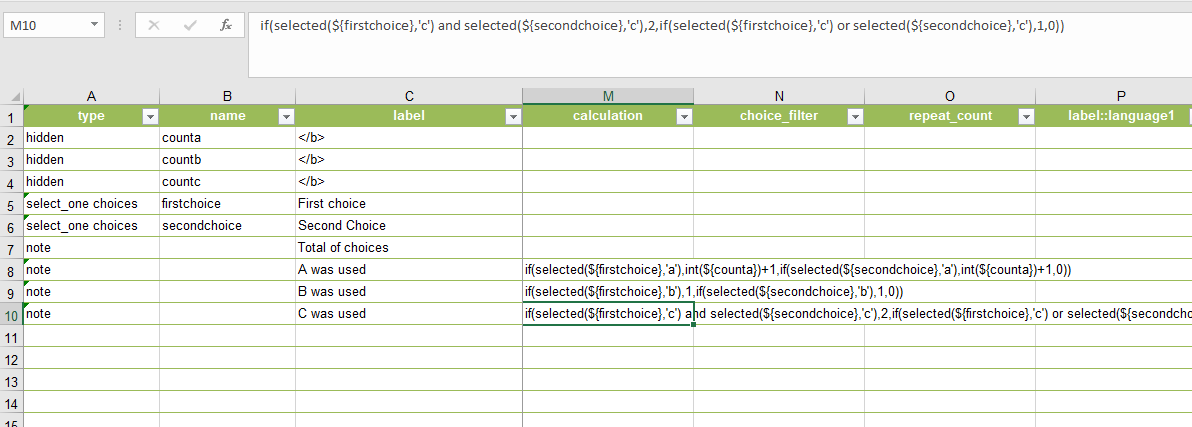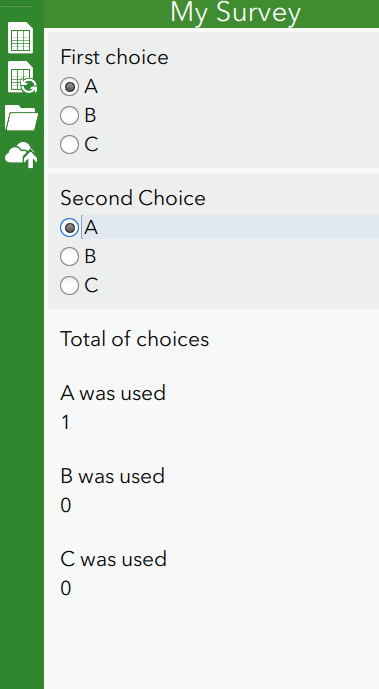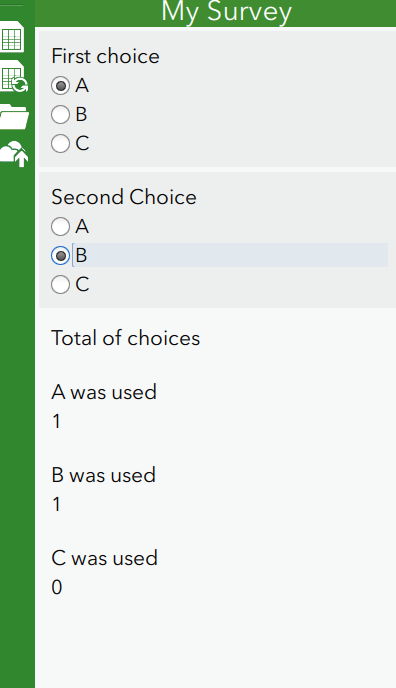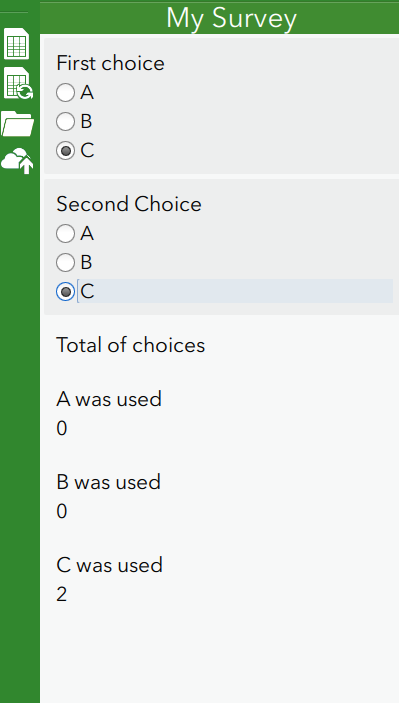# Count how many times a choice is used.

259
1
Jump to solution
03-28-2019 01:42 PMby
New Contributor II

Hi,

I'm trying to count the number of times a choice has been selected across many select_one questions.

as an exampleThe count works when only one instance is chosen, but fails to add values.

I realise this is due to the use of the 'if' statement as a control.

However if i use

if(selected(\${firstchoice,'a') and selected(\${secondchoice},'a'),2,if(selected(\${firstchoice},'a') or selected(\${secondchoice},'a'),1,0) it does work. (used for option 'c' above)

This solution is okay if there are only 2 options, however I want to count the number across 23 questions and the if statement would have 100's of permutations to enter.

There must be a better way.

I have tried using hidden fields as variable to hold the count, which doesn't work, (as in the first note field above), but i cant get the addition to work.

I have tried using countif but that is not a recognised function, and count(selected()) only works across multiple selects which is not an option for my audit

Any ideas?

1 Solution

Accepted Solutionsby
New Contributor II

I've solved it...

simply used an if statement to assess which option is selected then added up each answer

if(selected(\${firstchoice},'a'),1,0)+if(selected(\${secondchoice},'a'),1,0)

if(selected(\${firstchoice},'b'),1,0)+if(selected(\${secondchoice},'b'),1,0)

if(selected(\${firstchoice},'c'),1,0)+if(selected(\${secondchoice},'c'),1,0)

rookie mistake.....tried to over complicate it1 Replyby
New Contributor II

I've solved it...

simply used an if statement to assess which option is selected then added up each answer

if(selected(\${firstchoice},'a'),1,0)+if(selected(\${secondchoice},'a'),1,0)

if(selected(\${firstchoice},'b'),1,0)+if(selected(\${secondchoice},'b'),1,0)

if(selected(\${firstchoice},'c'),1,0)+if(selected(\${secondchoice},'c'),1,0)

rookie mistake.....tried to over complicate it